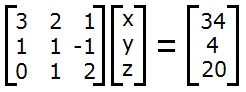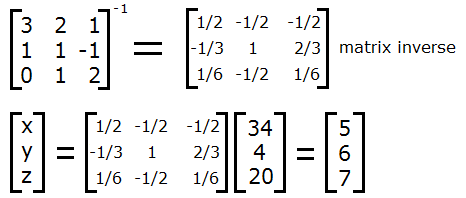# How to Solve a System of Three Equations in Three Variables

 X + Y + Z   = X + Y + Z   = X + Y + Z   =

In linear algebra, there are many methods for solving systems of linear equations in several variables. The simplest way is to apply substitution to eliminate the variables one by one. A more advanced technique is to use matrices. Both methods can be applied to systems with more than three variables. You can also use the system of equations calculator on the left. Simply input the coefficients and constant terms, and the calculator will output the unique solution set if one exists.

#### Substitution

To solve a system of three linear equations in three variables by substitution, you must first identify the easiest equation to work with. For example, suppose the three equations are

3x + 2y + z = 34
x + y - z = 4
y + 2z = 20

The easiest equation to work with is the last one. If we solve the equation for y, we get y = 20 - 2z. Now we plug this expression into the first two equations:

3x + 2(20-2z) + z = 34    or    3x - 3z = -6
x + (20-2z) - z = 4          or    x - 3z = -16

Of these two equations, the first one can be reduced to x = -2 + z. If we plug that expression into the second equation, we get

(-2+z) - 3z = -16, or
z = 7.

Using the solution z = 7, we can work backwards to find y = 6 and x = 5.

Sometimes the method of substitution will present equations that cannot be solved. For example, if you encounter a tautological equation such as x = x or 2 = 2 during the process, it means that the system of equations does not have a unique solution set, but rather infinitely many solutions. If you do the algebra and end up with a false equation such as 2 = 3, it means that the system has no solutions. Examples:

x + y + z = 12
2x + y - z = 20
3x + 2y = 32
This system has infinitely many solutions

x + y + z = 12
2x + y - z = 20
3x + 3y + 3z = 28
This system has no solution

#### Matrices

To solve a system of equations with matrix algebra, you must create a 3 by 3 matrix out of the coefficients of the equations, and 3 by 1 matrix out of the constant terms. For example, if we use the very first example of a system of equations, we can set up the matrix equationSince the 3 by 3 matrix in this problem has a determinant equal to 6, the matrix is invertible. (When the determinant is zero, the matrix is not invertible and no unique solution exists.) Then you can use the matrix inverse to find the solution for x, y, and z:So you end up with x = 5, y = 6, and z = 7 as before.